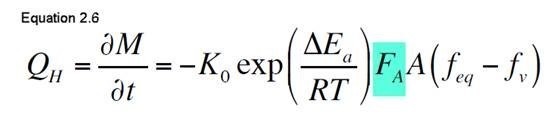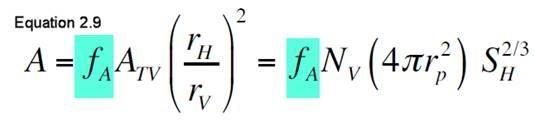0I am currently doing some simulations of core based experiments using the kinetic model.

With regards to terms in the equations I would like to confirm the notation.

As these equations have been shared in multiple works, I will refer only  to the HYDRATE v1.5 OPTION OF TOUGH+ v1.5 manual.

Equation (2.6) describes the behavior of hydrate mass over time, based on Kim model.

The F_A  term is defined as an area adjustment factor.This factor corresponds to the third parameter that can be set by the user at the HYDRATE section, line 12.

However, when it comes to the calculation of the  term in equation 2.6, which is the surface area participating in the reaction, I encounter that there is also an area adjustment factor, this time represented by f_A in equation (2.9).Is this f_A  term in equation 2.9, the same as  in equation 2.6?